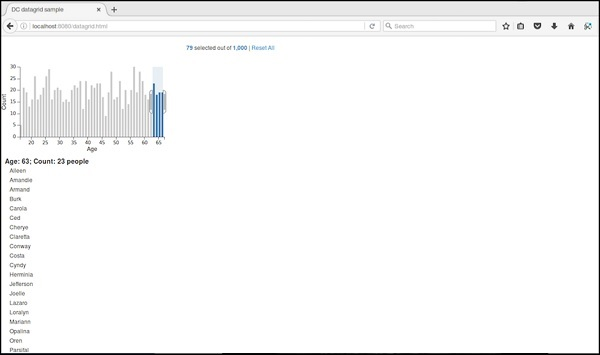# DC.js - Data Grid

Data grid is used to filter and display the records. This chapter explains about data grid in detail.

## Data Grid Methods

Before moving on to draw a data grid, we should understand the dc.dataGrid class and its methods. This class uses a mixin to get the basic functionality of drawing a data grid chart, which is defined below −

• dc.baseMixin

The dc.dataGrid gets all the methods of this mixin as well as has its own methods to draw the data grid, which is explained below −

### beginSlice( [slice])

This method is used to get or set the index of the beginning slice. This method is useful when implementing pagination.

Similarly, you can perform endSlice().

### group(function)

This method is used to perform the group function for the data grid.

### html( [html])

This method is used to get or set the function to generate a dynamic html.

### order( [order])

It is used to sort the order function.

### size( [size])

It is used to display the number of items in the grid.

### sortBy( [sortByFunction])

This method is used to get or set the sort-by function. We can sort a particular field using this function. For example: we can sort by age, which is defined below −

```chart.sortBy(function(d) {
return d.age;
});
```

## Data Grid Example

Let us perform a data grid in DC. To do this, we need to follow the steps given below −

Let us add styles in CSS using the coding below −

```.dc-chart { font-size: 12px; }
.dc-grid-top { padding-left: 10px; font-size: 14px; font-weight: bold; }
.dc-grid-item { padding-left: 10px; font-size: 12px; font-weight: normal; }
```

Here, we have assigned the styles for the chart, grid-top and grid-item.

### Step 2: Create a variable

Let us create a variable in DC as explained below −

```var barChart = dc.barChart('#line');
var countChart = dc.dataCount("#mystats");
var gridChart = dc.dataGrid("#mygrid");
```

Here, we have assigned a barChart variable id in line, countChart id is mystats and gridChart id is mygrid.

### Step 3: Read the data

Read the data from the people.csv file as shown below −

```d3.csv("data/people.csv", function(errors, people) {
var mycrossfilter = crossfilter(people);
}
```

If data is not present, then it returns an error. Now, assign the data to a crossfilter.

Here, we used the same people.csv file, which was used in our previous charting examples. It looks like as shown below −

```id,name,gender,DOB,MaritalStatus,CreditCardType
1,Damaris,Female,1973-02-18,false,visa-electron
2,Barbe,Female,1969-04-10,true,americanexpress
3,Belia,Female,1960-04-16,false,maestro
4,Leoline,Female,1995-01-19,true,bankcard
5,Valentine,Female,1992-04-16,false,
6,Rosanne,Female,1985-01-05,true,bankcard
7,Shalna,Female,1956-11-01,false,jcb
8,Mordy,Male,1990-03-27,true,china-unionpay

..........................................
.........................................
```

### Step 4: Set the dimension

You can set the dimension using the coding given below −

```var ageDimension = mycrossfilter.dimension(function(data) {
return ~~((Date.now() - new Date(data.DOB)) / (31557600000))
});
```

After the dimension has been assigned, group the age using the coding given below −

```var ageGroup = ageDimension.group().reduceCount();
```

### Step 5: Generate a chart

Now, generate a bar chart using the coding given below −

```barChart
.width(400)
.height(200)
.x(d3.scale.linear().domain([15,70]))
.yAxisLabel("Count")
.xAxisLabel("Age")
.elasticY(true)
.elasticX(true)
.dimension(ageDimension)
.group(ageGroup);
```

Here,

• We have assigned the chart width as 400 and height as 200.
• Next, we have specified the domain range as [15,70].
• We have set the x-axis label as age and y-axis label as count.
• We have specified the elasticY and X function as true.

### Step 6: Create the grid chart

Now, create the grid chart using the coding given below −

```gridChart
.dimension(ageDimension)
.group(function (data) {
return ~~((Date.now() - new Date(data.DOB)) / (31557600000));
})
```

### Step 7: Render the grid

Now, render the grid using the coding given below −

```.size(100)
.htmlGroup (function(d) {
return 'Age: ' + d.key +
'; Count: ' + d.values.length +
' people'
})
.html (function(d) { return d.name; })
.sortBy(function (d) {
return d.name;
})
.order(d3.ascending);

barChart.render();
countChart.render();
gridChart.render();
```

Here, we have sorted the name using the html() function and have finally rendered the chart.

### Step 8: Working example

The complete code is as follows. Create a webpage datagrid.html and add the following changes to it.

```<html>
<title>DC datagrid sample</title>
<link rel = "stylesheet" type = "text/css" href = "css/bootstrap.css">
<link rel = "stylesheet" type = "text/css" href = "css/dc.css"/>

<style>
.dc-chart { font-size: 12px; }
.dc-grid-top { padding-left: 10px; font-size: 14px; font-weight: bold; }
.dc-grid-item { padding-left: 10px; font-size: 12px; font-weight: normal; }
</style>

<script src = "js/d3.js"></script>
<script src = "js/crossfilter.js"></script>
<script src = "js/dc.js"></script>

<body>
<div>
<div style = "width: 600px;">
<div id = "mystats" class = "dc-data-count" style = "float: right">
<span class = "filter-count"></span> selected out of <span
class = "total-count"></span> | <a href = "javascript:dc.filterAll();
dc.renderAll();">Reset All</a>
</div>
</div>

<div style = "clear: both; padding-top: 20px;">
<div>
<div id = "line"></div>
</div>
</div>

<div style = "clear: both">
<div class = "dc-data-grid" id = "mygrid"></div>
</div>
</div>

<script language = "javascript">
var barChart = dc.barChart('#line');
var countChart = dc.dataCount("#mystats");
var gridChart = dc.dataGrid("#mygrid");

d3.csv("data/people.csv", function(errors, people) {
var mycrossfilter = crossfilter(people);

// age dimension
var ageDimension = mycrossfilter.dimension(function(data) {
return ~~((Date.now() - new Date(data.DOB)) / (31557600000))
});
var ageGroup = ageDimension.group().reduceCount();

barChart
.width(400)
.height(200)
.x(d3.scale.linear().domain([15,70]))
.yAxisLabel("Count")
.xAxisLabel("Age")
.elasticY(true)
.elasticX(true)
.dimension(ageDimension)
.group(ageGroup);

countChart
.dimension(mycrossfilter)
.group(mycrossfilter.groupAll());

gridChart
.dimension(ageDimension)
.group(function (data) {
return ~~((Date.now() - new Date(data.DOB)) / (31557600000));
})
.size(100)
.htmlGroup (function(d) {
return 'Age: ' + d.key +
'; Count: ' + d.values.length +
' people'
})
.html (function(d) { return d.name; })
.sortBy(function (d) {
return d.name;
})
.order(d3.ascending);

barChart.render();
countChart.render();
gridChart.render();
});
</script>
</body>
</html>
```

Now, request the browser and we will see the following response.

Initially, the grid chart looks like the following screenshot.

If you select a particular age between 63 and 66, it filters out the following records.